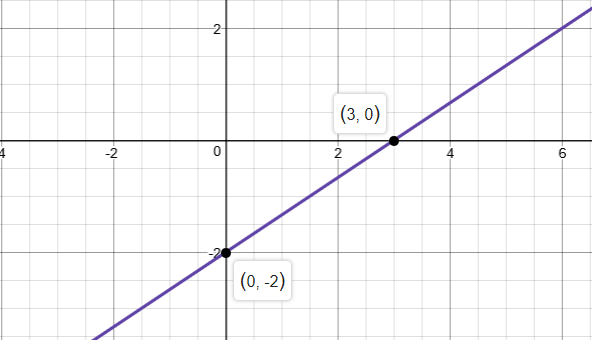# Graph equation 2x – 3y = 6

We thoroughly check each answer to a question to provide you with the most correct answers. Found a mistake? Let us know about it through the REPORT button at the bottom of the page.

Graph equation: 2x – 3y = 6

We can graph an equation by determining the x- and y- intercepts and then drawing the line through them.

Set x = 0 to find the y-intercept

2*0 – 3y = 6

0 – 3y = 6

y = -2

Set y = 0 to find the x-intercept

2x – 3*0 = 6

2x – 0 = 6

x = 3